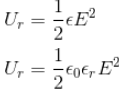# RBSE Solutions For Class 12 Physics Chapter 2: Gauss's Law and its Applications | Textbook Important Questions & Answers

The answers are provided for all the questions of Chapter 2 Physics of RBSE Class 12. Students can go through these questions to understand the concepts better and score well in the board examination and entrance examinations for various professional courses. These solutions are provided by the team of experts to provide the best and accurate solutions to the questions. Do check BYJU’S RBSE Class 12 solutions page to get the answers for textbook questions of all subjects.

## Multiple Choice Type Questions

Q1: Electric-field intensity is maximum due to a uniformly charged non-conducting

Sphere

1. At the centre
2. Between a point on the surface and the centre
3. At surface
4. At infinity

Q2: A charge of 1pC is placed at the centre of a cube of side 0.2m.The electric flux leaving each vertex of the cube in V/m is

1. 1.12 x 104
2. 2.2 x 104
3. 1.88 x 104
4. 3.14 x 104

Q3: On giving a negative charge to a soap bubble, its radius

1. Decreases
2. Increases
3. Remain unchanged

Q4: A charge q is in a sphere and the flux leaving out is q/Є0. How much would be the change in the electric flux on reducing the radius to its half?

1. Becomes 4 times the initial value
2. Becomes ¼ the initial value
3. Becomes half of the initial value
4. Remains unchanged

Q5: The radii of two conducting spheres are a and b. They are charged by an equal amount then the ratio of electric field intensity at its surface

1. b2: a2
2. 1:1
3. a2: b2
4. b: a

Q6: The radii of two conduction spheres are a and b. They are charged by equal charge density. What would be the ratio of the electric field intensities at their surface?

1. b2: a2
2. 1:1
3. a2: b2
4. b : a

## Very Short Answer Type Questions

Q1: When does the electric flux through an area element placed in the electric field be zero?

Answer: The electric flux will be zero when the area element is at 90 degrees with the electric field.

Q2: At what positions the electric field intensity due to a uniformly charged sphere is zero?

Answer: The electric field intensity due to the uniformly charged sphere is zero at the centre of sphere and at infinity

Q3: Write the expression for force per unit area of a charged and give its direction.

Answer: The value of force F=σ2 /2Є0 and it is directed normally outward

Q4: Where does the energy due to a charge is stored?

Answer: The energy is stored in the region of the electric field

Q5: A charge Q is given to a conducting sphere of diameter d what is the value of an electric field inside the sphere?

Answer: The value of the electric field inside the sphere will be zero

Q6: Suppose the Coulomb’s Law has a 1/r3 dependence instead of dependence 1/r2 is Gauss’s law still valid?

Answer: The Gauss law will be valid only for 1/r2

Q7: If the net charge enclosed by a Gaussian surface is positive then what is the nature of flux through the surface?

Answer: The flux will be positive

Q8: If the net flux through some closed surface in an electric field is zero what can be said about the surface?

1. Total charge enclosed by the system is zero
2. Total flux entering the surface=Total flux leaving the surface

Q9: If the net charge enclosed by a Gaussian surface is zero does it mean that the electric field at every point on the surface is zero?

Answer: No, it is not necessary that the electric field will be zero

This situation is only possible only when the electric field and area are perpendicular to each other

Q10: Define linear charge density.

Answer: Linear charge density is the amount of electric charge per unit length

Q11: What will be the change in electric field in moving from one side to the other of a charged plane sheet having a surface charge density σ.

Answer: The change in Electric field = E1 – E2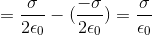Q12: Graph the variation of the electric field with distance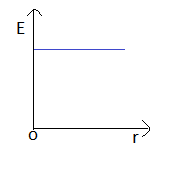Q13: What is the value of the electric field at the centre of a uniformly charged non conducting sphere?

Answer: The electric field at the centre of the electric field will be zero since the charge inside the sphere will be zero

Q14: A charge q is at the centre of a sphere. If now this charge is placed at the centre of a cylinder of the same volume then what will be the ratio of net flux in the two cases

Answer: The electric flux will be equal for both cases

The ration is given by 1 : 1

Q1: Explain the term electric flux. Write its SI unit and dimensions

Answer: The total number of electric field lines passing a given area in a unit time is defined as the electric flux.

It the plane is normal to the flow of the electric field then the value of the electric field is given by

Ф =EA

When the same plane is tilted at an angle Ɵ, the projected area is given as AcosƟ and the total flux through this surface is given as

Ф = EAcosθ

The SI unit for flux is given by volt metre (V m)

Q2: Explain the term linear charge density. Write its SI unit.

Answer: The charge density tells us how much charge is stored in a particular field.

Linear charge density is the charge per unit length and it is represented by λ

λ=q/l

where q is the charge and l is the length over which it is distributed

S.I unit of Linear charge density is coulomb/m

Q3: Explain the term surface charge density. Write its SI unit

Answer: The charge per unit area is called the surface charge density and it is represented as σ

σ =q/A

where q is the charge and A is the area over which it is distributed

S.I unit of Linear charge density is coulomb/m3

Q4: Explain the term volume charge density. Write its SI unit.

Answer: The charge per unit volume is called the volume charge density and it is represented as ρ

ρ =q/A

where q is the charge and V is the volume over which it is distributed

S.I unit of Linear charge density is coulomb/m3

Q5: State Gauss’s law for electrostatics

Answer: According to the Gauss law, the total flux linked with a closed surface is 1/ε0 times the charge bounded by the closed surface.

Mathematically Gauss Law can be written as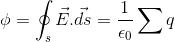Where є0 is the permittivity of free space and is the total charge in the surface

Q6: The excess charge given to a conductor resides always on its outer surface? Why?

Answer: The electric field inside a charged sphere is zero (E=0). Therefore, the emergent flux ( Φ) from the Gaussian surface is 0.

Electric flux is given by Φ=q/Є0

Therefore Φ=q/Є0=0

q=ΦЄ0=0

Which means the charge inside the conductor is zero and the charges reside on the outer surface

Q6. Verify Gauss’s law from Coulomb’s law

Answer: Let us derive the Gauss’s theorem by using Coulomb’s Law

According to Coulomb’s law the intensity of electric field (E) at a distance r from charge q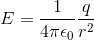The electric flux is given by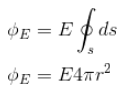Because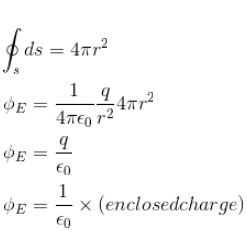This is Gauss’s Theorem

Q7: You are travelling in a car. Lightning is expected, what should you do about your safety?

Answer: To stay safe in the car we should close the windows of the car. Closing the window makes sure the charges lie on the surface of the car.

Q8: The charge densities on parallel bases of two infinite expansions are +σ and -σ respectively. What would be the electric field intensities at a point between them?

In the points between both the plates, the electric field produced due to both plates will be in the same direction.

The resultant electric field is given by

E = E1+E2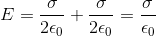## Essay Type Questions

Q1: Establish expressions for electric force and electrostatic pressure on the surface of a charged conductor?

Answer: The excess charges given to the surface recedes on the surface of the conductor. There is a force of repulsion acting between the charges. Thus, the net force on the surface is the vector sum of force acting on all the small elements.

Let us consider the surface charge density on the surface of the conductor to be σ. Two points are considered inside and outside the surface. Let the point outside the surface be P1 and the surface inside is P2.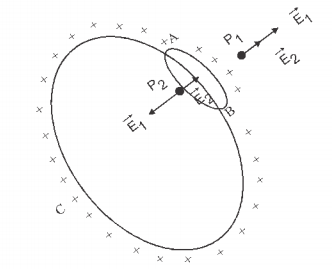The electric field outside the charged conductor is given by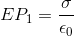—————————————–(1)

The electric field inside the conductor is zero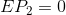——————————————-(2)

Now we are dividing the conductor into two parts in order to understand the repulsive force between the elements.

(i) A small element AB whose surface is ds

(ii) The remaining part of the conductor ACB

If E1 and E2 are the electric fields due to AB and ACB, respectively at points near the surface.

EP1= E1+E——————————–(3)

(E1 and E2 are in the same direction at the point P1)

EP2= E1+E2———————————(4)

(E1 and E2 are in the opposite direction at P2)

From equations (2) and (4) we have

E1 – E2=0

i.e.,E1 = E2——————————————-(5)

From equations (1),(3) and (5)

E2+E2=σ/ε0

E2 =σ/2ε0

E2 is the electric field intensity at element AB due to the element ACB. If the total charge on the element AB is dq , then the force on element dF = E2dq

That can be written as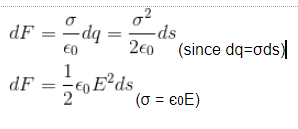Force acting on the complete surface is given by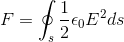The force per unit area also known as pressure is given by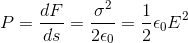Q2: Establish expression for energy stored per unit volume in the electric field

Answer: In order to increase the charge on a conductor or to increase the volume of the volume the region work has to be done. The work done gets stored as energy in the electric field.

Let us take the example of the spherical shell of radius r with a surface charge density σ.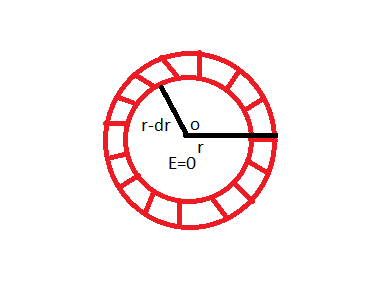Outward pressure on the surface of the shell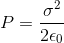So the outward force on the surface is

F = (Pressure)(Area)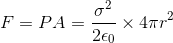The work done against this force in compressing the shell by a small amount dr is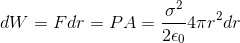The decrease in the volume of the sphere due to compression

dV=4πr2dr

Thus,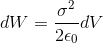Energy stored in the whole system due to electric field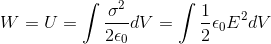The energy stored per unit volume in the electric field or energy density.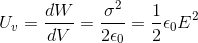It there is any other medium in place of vacuum or air, then